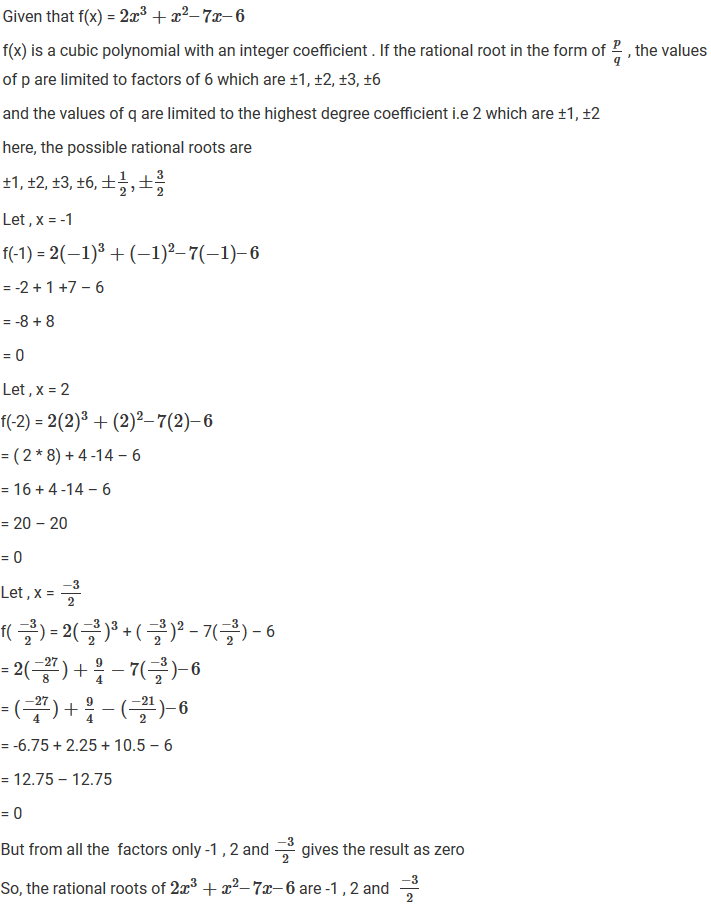#### Chapter 6 Factorization of Polynomials R.D. Sharma Solutions for Class 9th Math Exercise 6.2

Exercise 6.2

1. If f(x) = 2x3-13x2+17x+12, find
(i) f(2)
(ii) f(-3)
(iii) f(0)

Solution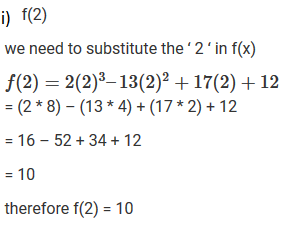2. Verify whether the indicated numbers are zeros of the polynomials corresponding to them in the following cases:
(i) f(x) = 3x+1, x=-1/3
(ii) f(x) = x2-1, x=1,-1
(iii) g(x) = 3x2-2, x=2/√3, -2/√3
(iv) p(x) =  x3-6x2+11x-6, x=1,2,3
(v) f(x) =5x-π, x=4/5
(vi) f(x) = x2, x =0
(vii) f(x) = lx+m, x=m/1
(viii) f(x) = 2x+1, x=1/2

Solution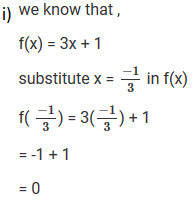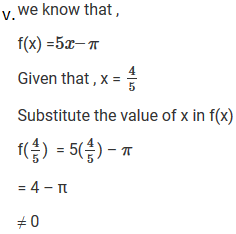3. If x=2 is a root of the polynomial f(x) =2x2-3x+7a, find the value of a.
Solution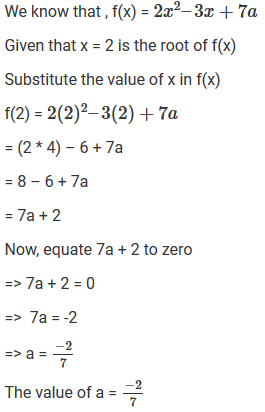4. If x=-1/2 is a zero of the polynomial p(x) = 8x2-ax2+2, find the value of a.
Solution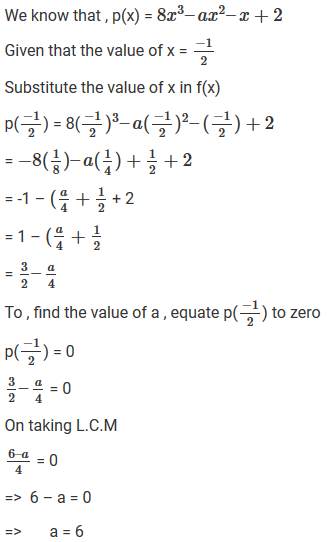5. If x=0 and x=-1 are the roots of the polynomial f(x) = 2x3-3x2+ax+b, find the value of a and b.
Solution6. Find the integral roots of the polynomial f(x) =  x3+6x2+11x+6
Solution7. Find rational of the polynomial f(x) = 2x3+x2-7x-6
Solution